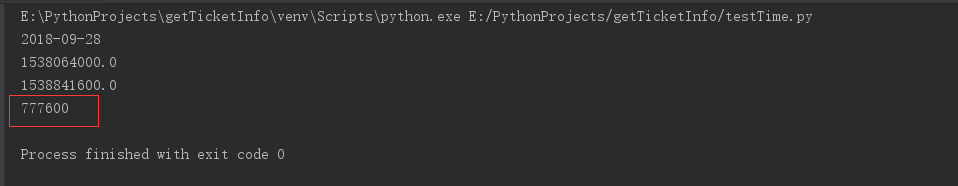python3 比较数字大小
2019-02-22 09:37:22 archsmart 阅读数 836
``````num1 = input("num1 ")
num2 = input("num2 ")
num3 = input("num3 ")
max_num = 0
if num1>num2:
max_num=num1
if max_num>num3:
print "The max num is",max_num
else:
print "The max num is",num3
else:
max_num=num2
if max_num>num3:
print "The max num is",max_num
else:
print "The max num is",num3
``````
2018-02-12 11:01:00 weixin_34326558 阅读数 172
``````1、python的比较总是检查复合对象的所有部分，直到可以得出结果为止。
2、会自动遍历嵌套的所有数据结构，有多深走多深，首次发现的差值将决定比较的结果
3、== :操作符测试值的相等性
4、is :表达式测试对象的一致性，表示同一个对象，在同一内存地址中

python对象的大小比较规则：

1、数字通过相对大小进行比较
2、字符串按照字典顺序（编码顺序）,一个字符接一个字符进行比较
3、列表和元组从左到右对每部分的内容进行比较
4、字典通过排序后的(键,值)列表进行比较，pyton3.0中不支持
5、数字混合类型pyton3.0中不支持，拼音。6中允许
```` ````
2018-04-09 15:06:25 pansanday 阅读数 2466

ex3.py

```print("I will now count my chickens:")

print('Hens', 25 + 30 / 6)

print("Roosters", 100 - 25 * 3 %4)

print("Now I will count the eggs:")

print(3+2+1-5+4%2-1/4+6)

print("Is it true that 3+2<5-7?")

print(3+2< 5-7)

print("What is 3 + 2?", 3+2)

print("What is 5 - 7?", 5-7)

print("Oh, that's why it's false.")

print("Is it greater?", 5 > -2)

print("Is it greater or equal?", 5 >= -2)

print("Is it less or equal?", 5 <= -2)```

```\$ python3 ex3.py
I will now count my chickens:
Hens 30.0
Roosters 97
Now I will count the eggs:
6.75
Is it true that 3+2<5-7?
False
What is 3 + 2? 5
What is 5 - 7? -2
Oh, that's why it's false.
Is it greater? True
Is it greater or equal? True
Is it less or equal? False```

2018-03-20 13:59:23 weixin_41869526 阅读数 991

a=input('>>>')

b=input('>>>')

a=int(a)

b=int(b)

if a>b：

print (a)

else:

print(b)

或者

print(max(a,b))

val=input（'>>')

val=int(val)

if val >= 1000:   #fold

if val >= 10000:

print(5)

else:

print(4)

else:

if val >=100:

print(3)

elif val >=10:

print(2)

else:

print(1)

2018-09-28 23:04:48 HoWaterSuper 阅读数 3195

#str转time

`time.strptime('2018-09-28',"%Y-%m-%d")`

#str转datetime

datetime.datetime.striptime('2018-09-28',"%Y-%m-%d")

#time 转string

`time.strftime("%y-%m-%d",time.localtime())`

``````import datetime
import time
#将日期字符串转为时间再比较，time，datetime,str
def valid_date(timestr):
#获取当前时间日期
nowTime_str = datetime.datetime.now().strftime('%Y-%m-%d')
print(nowTime_str)
#mktime参数为struc_time,将日期转化为秒，
e_time = time.mktime(time.strptime(nowTime_str,"%Y-%m-%d"))
print(e_time)
try:
s_time = time.mktime(time.strptime(timestr, '%Y-%m-%d'))
print(s_time)
#日期转化为int比较
diff = int(s_time)-int(e_time)
print(diff)
if diff >= 0:
return 1
else:
print('所查日期不能小于当前时间！！！')
return 0
except Exception as e:
print(e)
return 0
if __name__=='__main__':
valid_date("2018-10-07")``````python比较时间大小

a='2017-10-1822:17:46'b='2017-10-1922:17:40'printa>b#结果False解释：python中字符串的大小比较，是按照字符顺序，从前往后依次比较字符的ASCII数值，例如‘abc’要小于‘abd’。因此，时间字符串也可以直接比大小。

python等值和大小比较

python对象的比较（is，==,对象大小比较>,

#一、python对象的"==、is"比较"""1、python的比较总是检查复合对象的所有部分，直到可以得出结果为止。2、会自动遍历嵌套的所有数据结构，有多深走多深，首次发现的差值将决定比较的结果""""""==:操作符测试值的相等性is:表达式测试对象的一致性，表示同一个对象，在同一内存地址中输出结果：TrueFalse"""L1=[1,('A',2)]L2=

Python中字符和数字之间是怎么比较大小的？

Python 3（3）数据类型：数字

Python3入门专栏http://blog.csdn.net/column/details/19679.htmlPythonNumber数字python3的Number支持以下4种类型：bool布尔型：包含True和False两个值，python2没有bool值，而是用0，1来代替True，False；int整型：python3整型是没有限制大小的，可以当作Lo...